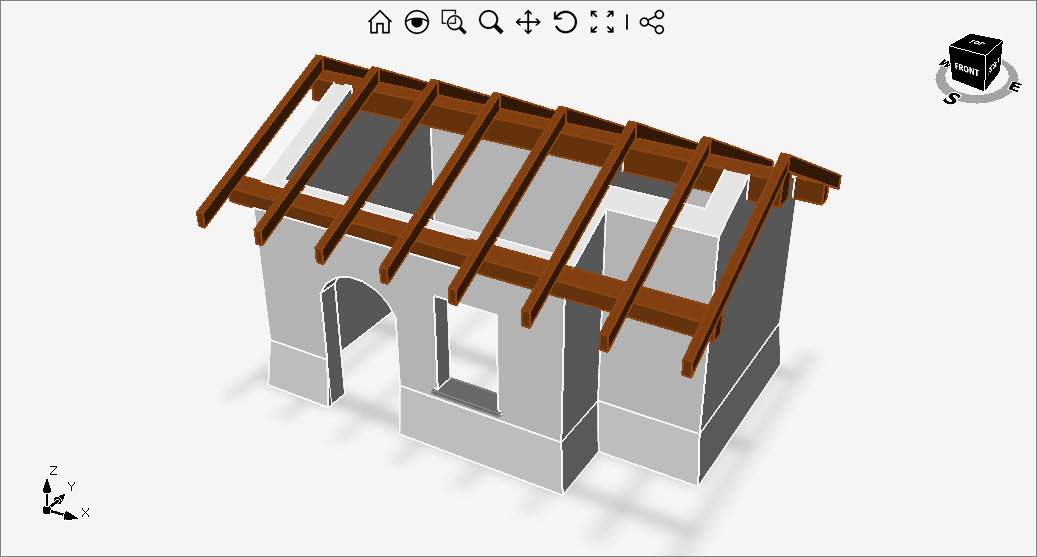# House

Use the following code to model with Solid entities an object like the one in the picture:``````var outer = Region.CreatePolygon(new Point3D[]
{
new Point3D(0, 0),
new Point3D(460, 0),
new Point3D(460, 100),
new Point3D(600, 100),
new Point3D(600, 400),
new Point3D(0, 400)
});

// House's extruded outer profile
Solid body = outer.ExtrudeAsSolid(400, 0);

// Big room at origin
Solid bigRoom = Solid.CreateBox(400, 340, 400);

// Moves big room in place
bigRoom.Translate(30, 30, 0);

// Cuts the big room from the house's body
Solid[] firstCut = Solid.Difference(body, bigRoom);

// Small room
Solid smallRoom = Solid.CreateBox(130, 240, 400);

// Moves small room in place
smallRoom.Translate(440, 130, 0);

// Cuts the small room from the house's body
Solid[] secondCut = Solid.Difference(firstCut, smallRoom);

// Draws the main door profile on a vertical plane
Plane pln = new Plane(new Point3D(100, 40, 0), Vector3D.AxisX, Vector3D.AxisZ);

Line l1 = new Line(pln, 0, 180, 0, 0);
Line l2 = new Line(pln, 0, 0, 120, 0);
Line l3 = new Line(pln, 120, 0, 120, 180);
Arc a1 = new Arc(pln, new Point2D(60, 155), new Point2D(120, 180), new Point2D(0, 180));

devDept.Eyeshot.Entities.Region reg = new devDept.Eyeshot.Entities.Region(new CompositeCurve(l1, l2, l3, a1));

// Cuts the main door profile from the house's body
secondCut.ExtrudeRemove(reg, 50, 1);

// central horizontal beam
Solid beam1 = Solid.CreateBox(680, 30, 40);

// moves in place
beam1.Translate(-40, 185, 360);

// cut the house's body
Solid[] thirdCut = Solid.Difference(secondCut, beam1);

// same for other two horizontal beams
Solid beam2 = Solid.CreateBox(680, 20, 40);

beam2.Translate(-40, 0, 280);

Solid[] fourthCut = Solid.Difference(thirdCut, beam2);

Solid beam3 = Solid.CreateBox(680, 20, 40);

beam3.Translate(-40, 380, 280);

Solid[] fifthCut = Solid.Difference(fourthCut, beam3);

// Intersection tool loop
outer = Region.CreatePolygon(Plane.YZ, new Point2D[]
{
new Point2D(0, 0),
new Point2D(400, 0),
new Point2D(400, 300),
new Point2D(200, 400),
new Point2D(0, 300)
});

// Tool body
Solid intersectionTool = outer.ExtrudeAsSolid(Vector3D.AxisX * 680, 0);

// Moves the tool in place
intersectionTool.Translate(-40, 0, 0);

// Intersects the house's body with the tool
Solid[] firstInters = Solid.Intersection(fifthCut, intersectionTool);

// Intersects the horizontal beams with the tool
Solid[] secondInters = Solid.Intersection(beam1, intersectionTool);
Solid[] thirdInters = Solid.Intersection(beam2, intersectionTool);
Solid[] fourthInters = Solid.Intersection(beam3, intersectionTool);

// Adds beams to the scene

// Basement sweep rail
LinearPath rail = new LinearPath(new Point3D[]
{
new Point3D(220, 0),
new Point3D(460, 0),
new Point3D(460, 100),
new Point3D(600, 100),
new Point3D(600, 400),
new Point3D(0, 400),
new Point3D(0, 0),
new Point3D(100, 0)
});

// Basement sweep section
var section = Region.CreatePolygon(new Point3D[]
{
new Point3D(220, 0, 0),
new Point3D(220, -7.5, 0),
new Point3D(220, 0, 75),
});

// Sweep solid
Solid basement = section.SweepAsSolid(rail, 0);

// Merges sweep with the house's body
Solid[] firstUnion = Solid.Union(firstInters, basement);

// Internal door
Solid door = Solid.CreateBox(30, 80, 210);

// Moves internal door in place
door.Translate(420, 140, 0);

// Cuts the internal door from the house's body
Solid[] sixthCut = Solid.Difference(firstUnion, door);

Solid beam10 = Solid.CreateBox(10, 120, 20);
beam10.Translate(430, 120, 210);

Solid[] seventhCut = Solid.Difference(sixthCut, beam10);

// Window
Solid window = Solid.CreateBox(90, 50, 140);

// Moves window in place
window.Translate(280, -10, 90);

// Cuts the window from the house's body
Solid[] eighthCut = Solid.Difference(seventhCut, window);

Solid windowLedge = Solid.CreateBox(100, 35, 5);
windowLedge.Translate(275, -5, 85);

Solid[] sixthCut3 = Solid.Difference(eighthCut, windowLedge);

// Oblique beam loop
var obliqueLoop = Region.CreatePolygon(Plane.YZ, new Point2D[]
{
new Point2D(200, 0),
new Point2D(-60, -130),
new Point2D(-60, -150),
new Point2D(200, -20)
});

// Oblique beam
Solid oblique = obliqueLoop.ExtrudeAsSolid(10, 0);

// Moves in place
oblique.Translate(-40, 0, 420);

// A list of entities we need to mirror
List<Entity> toBeMirrored = new List<Entity>();

// Copies and adds the oblique beam
for (int i = 0; i < 7; i++)
{

Entity clone = (Entity)oblique.Clone();

clone.Translate((((680 - 8 * 10) / 7.0) + 10) * (i + 1), 0, 0);

}

// Copies and mirrors
int count = toBeMirrored.Count;

Plane mirrorPlane = Plane.ZX;

mirrorPlane.Origin.Y = 200;

Mirror m = new Mirror(mirrorPlane);

for (int i = 0; i < count; i++)
{
Entity clone = (Entity)toBeMirrored[i].Clone();

clone.TransformBy(m);